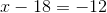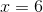## Example Questions

← Previous 1

### Example Question #1 : How To Find Out When An Equation Has No Solution

Given the following system, find the solution:

x = y – 2

2x – 2y = 2

(1, 2)

(0, 0)

(1, 1)

(0, 1)

no solution

no solution

Explanation:

When 2 equations in a system have the same slopes, they will either have no solution or infinite solutions. Since the y-intercepts are not the same, there is no solution to this system.

### Example Question #2 : How To Find Out When An Equation Has No Solution

Solve for: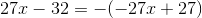No solution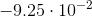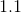Infinite Solutions

No solution

Explanation:Like other "solve for x" problems, to begin it, the goal is to get x by itself on one side of the equals sign. In this problem, before doing so, the imaginary -1 in front of (-27x+27) must be distributed.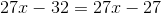Once this is done, you may start to try to get x by itself.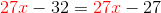However, when subtracting 27x from either side and doing the same on the other,  the 27x term cancels out. As a result, the equation becomes: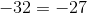We know this is an untrue statement because these numbers are 5 spaces away from each other on the number line. The final answer is No Solution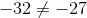### Example Question #3 : How To Find Out When An Equation Has No Solution

Given the following system, find the solution: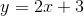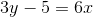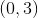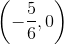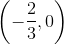No solution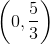No solution

Explanation:

When two equations have the same slope, they will have either no solution or infinite solutions.  By putting both equations into the form, we get:and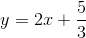With the equations in this form, we can see that they have the same slope, but different y-intercepts.  Therefore, there is no solution to this system.

### Example Question #4 : How To Find Out When An Equation Has No Solution

Solve the following equation for: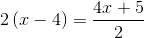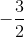Infinite solutionsNo solution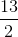No solution

Explanation:

In order to solve for, we must getby itself on one side of the equation.First, we can distribute theon the left side of the equal sign and theon the right side.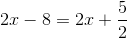When we try to getby itself, theterms on each side of the equation cancel out, leaving us with: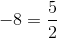We know this is an untrue statement, so there is no solution to this equation.

### Example Question #2 : How To Find Out When An Equation Has No Solution

Find the solution to the following equation if x = 3:

y = (4x2 - 2)/(9 - x2)

0

no possible solution

3

6

no possible solution

Explanation:

Substituting 3 in for x, you will get 0 in the denominator of the fraction. It is not possible to have 0 be the denominator for a fraction so there is no possible solution to this equation.

### Example Question #4 : How To Find Out When An Equation Has No Solution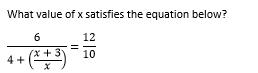–3

1

–1/2

3

There is no solution

There is no solution

Explanation: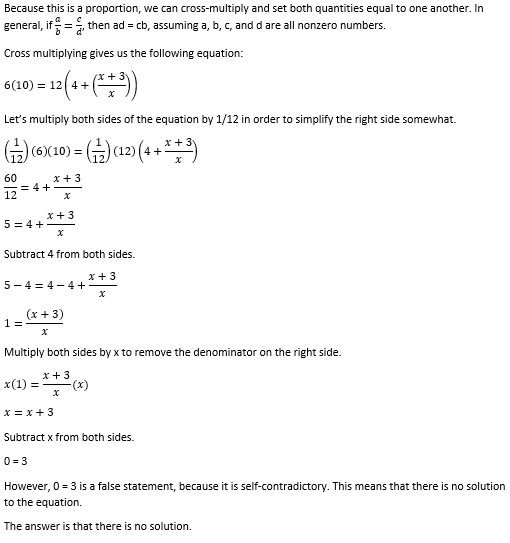### Example Question #2 : How To Find Out When An Equation Has No Solution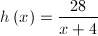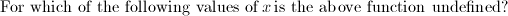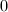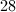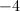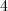Explanation:

A fraction is considered undefined when the denominator equals 0. Set the denominator equal to zero and solve for the variable.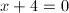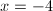### Example Question #8 : How To Find Out When An Equation Has No Solution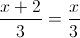Solve for.

No solutions.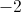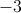No solutions.

Explanation:

Cross multiplying leaves, which is not possible.

### Example Question #1 : How To Find Out When An Equation Has No Solution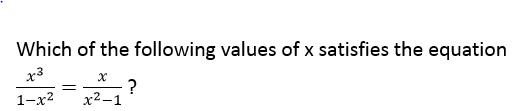I.  x = 0

II. x = –1

III. x = 1

I only

I, II, and III

II only

II and III only

III only

I only

Explanation: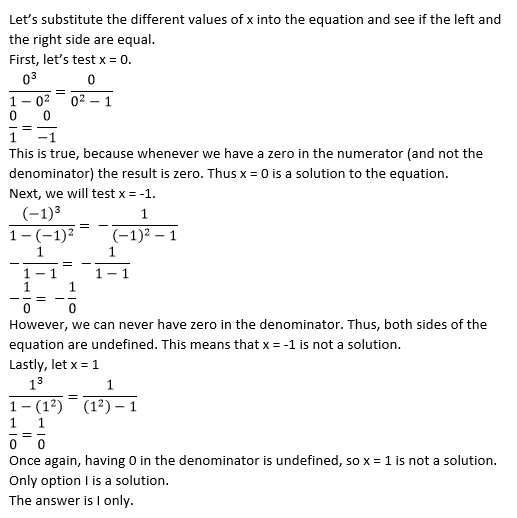### Example Question #10 : How To Find Out When An Equation Has No Solution

Solve: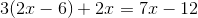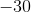Explanation:

First, distribute, making sure to watch for negatives.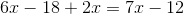Combine like terms.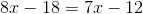Subtract 7x from both sides.